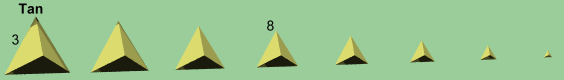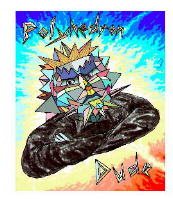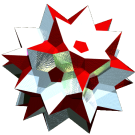Acronym tan
Name truncated penteract
Field of sections
`©`
wrt. pen
(5+4 sqrt(2))/sqrt(20) = 2.382945
wrt. tat
[1+sqrt(2)]/2 = 1.207107
Vertex figure
`©©`
Lace city
in approx. ASCII-art
 ```©   ``` ```o3x4x o3o4w o3o4w o3x4x -- o3o3x4x (tat) o3o4w o3o4w -- o3o3o4w (w-tes) o3o4w o3o4w -- o3o3o4w (w-tes) o3x4x o3o4w o3o4w o3x4x -- o3o3x4x (tat) ```
Coordinates ((1+sqrt(2))/2, (1+sqrt(2))/2, (1+sqrt(2))/2, (1+sqrt(2))/2, 1/2)   & all permutations, all changes of sign
Volume (1230+869 sqrt(2))/30 = 81.965053
Surface (505+360 sqrt(2)+sqrt(5))/3 = 338.784317
Dihedral angles
(at margins)
• at tet between pen and tat:   arccos[-1/sqrt(5)] = 116.565051°
• at tic between tat and tat:   90°
Confer
analogs:
truncated hypercube tCn
ExternalAs abstract polytope tan is isomorphic to quittin, thereby replacing octagons by octagrams, resp. tic by quith, resp. tat by quitit.

Incidence matrix according to Dynkin symbol

```o3o3o3x4x

. . . . . | 160 |   4  1 |   6  4 |   4  6 |  1  4
----------+-----+--------+--------+--------+------
. . . x . |   2 | 320  * |   3  1 |   3  3 |  1  3
. . . . x |   2 |   * 80 ♦   0  4 |   0  6 |  0  4
----------+-----+--------+--------+--------+------
. . o3x . |   3 |   3  0 | 320  * |   2  1 |  1  2
. . . x4x |   8 |   4  4 |   * 80 |   0  3 |  0  3
----------+-----+--------+--------+--------+------
. o3o3x . ♦   4 |   6  0 |   4  0 | 160  * |  1  1
. . o3x4x ♦  24 |  24 12 |   8  6 |   * 40 |  0  2
----------+-----+--------+--------+--------+------
o3o3o3x . ♦   5 |  10  0 |  10  0 |   5  0 | 32  *
. o3o3x4x ♦  64 |  96 32 |  64 24 |  16  8 |  * 10

snubbed forms: o3o3o3x4s
```